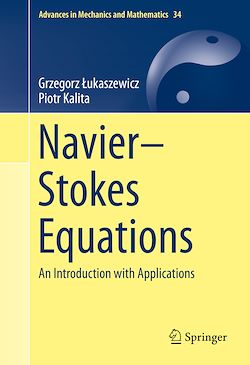# Navier–Stokes Equations

-English
390 Pages

Description

This volume is devoted to the study of the Navier–Stokes equations, providing a comprehensive reference for a range of applications: from advanced undergraduate students to engineers and professional mathematicians involved in research on fluid mechanics, dynamical systems, and mathematical modeling. Equipped with only a basic knowledge of calculus, functional analysis, and partial differential equations, the reader is introduced to the concept and applications of the Navier–Stokes equations through a series of fully self-contained chapters. Including lively illustrations that complement and elucidate the text, and a collection of exercises at the end of each chapter, this book is an indispensable, accessible, classroom-tested tool for teaching and understanding the Navier–Stokes equations.

Incompressible Navier–Stokes equations describe the dynamic motion (flow) of incompressible fluid, the unknowns being the velocity and pressure as functions of location (space) and time variables. A solution to these equations predicts the behavior of the fluid, assuming knowledge of its initial and boundary states. These equations are one of the most important models of mathematical physics: although they have been a subject of vivid research for more than 150 years, there are still many open problems due to the nature of nonlinearity present in the equations. The nonlinear convective term present in the equations leads to phenomena such as eddy flows and turbulence. In particular, the question of solution regularity for three-dimensional problem was appointed by Clay Institute as one of the Millennium Problems, the key problems in modern mathematics. The problem remains challenging and fascinating for mathematicians, and the applications of the Navier–Stokes equations range from aerodynamics (drag and lift forces), to the design of watercraft and hydroelectric power plants, to medical applications such as modeling the flow of blood in the circulatory system.

Subjects

##### Differential equation

Informations